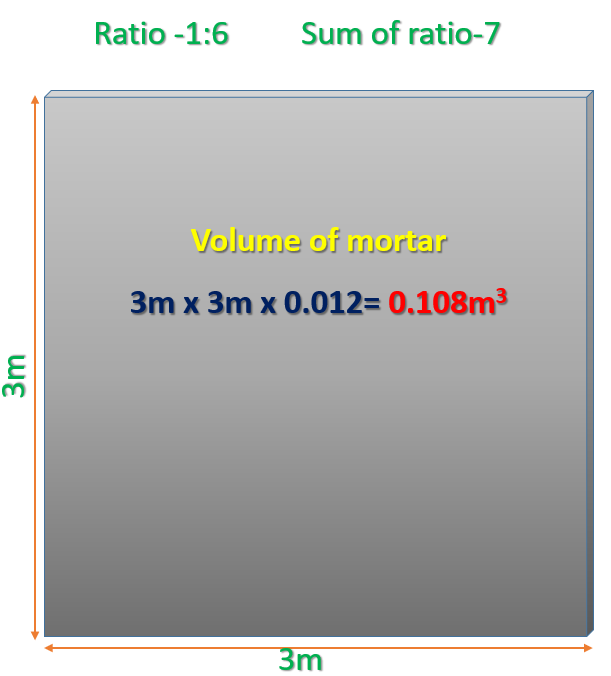# How to Calculate Cement, Sand Quantity for Plastering?

## Mortar Calculation for Plastering work

### Point Should Be Know Before Estimating

Density of Cement                         = 1440 kg/m3

Sand Density                                   = 1450-1500 kg/m3

Density of Aggregate                   = 1450-1550 kg/m3

How many KG in 1 bag of cement  = 50kg

Cement quantity  in litres in 1 bag of cement     = 34.7 litres

1 Bag of cement in  cubic metres     = 0.0347 cubic meter

How many CFT (Cubic Feet)        = 1.226 CFT

Numbers of Bags in 1 cubic metre cement = 28.8 Bags

The specific gravity of cement           = 3.15

Grade of cement                            = 33, 43, 53

Where 33, 43, 53 compressive strength of cement in N/mm2

—————————————————————————————————————————————————————–

Area of brick wall for plaster = 3m  3m =9m2Plaster Thickness = 12mm (Outer-20mm, Inner 12mm)
Volume of mortar = 9m2 x 0.012m            = 0.108m3
Ratio for Plaster Taken is                               = 1 : 6
Sum of ratio is                                                   = 7

Calculation for Cement Volume

Dry Volume of Mortar = 0.108 x 1.35 = 0.1458 m3

Cement= (1/7) x 0.1458 = 0.0208 m3

Density of Cement is 1440/m3

= 0.0208 x 1440              = 29.99 kg

We know each bag of cement is 50 kg

= (29.99/50)        = 0.599 Bags

Calculation for Sand Volume

Sand = (6/7) x 0.1458      = 0.124m3

The density of Sand is 1450/m3

= 0.0208 x 1450                = 181.2 kg

Now we find how many CFT (Cubic feet) Required

As we know that 1m3 = 35.31 CFT

= 0.124*35.31

= 4.37 CFT (Cubic Feet)Visit ourChannel for more information.

Tutorials Tips

### 31 Responses

1.PRASHANT JOGDAND says:

CALCULATOR FOR STEEL QTY

2.Paul A. Subah says:

good

•Eze miracle says:

Nice one i really learn something crucial here..

3.iswar says:

excellent, sir
awesome

•Tutorial Tips Civil says:

thank you

4.Alidu Alih says:

The calculation is amazing; it quiet comprehensible, I really appreciate. Thanks.

5.Sendil nathan says:

How to calculate the steel? Steel calculation formula =( d×d)/164 . Example suppose d= 8mm , sol = 64/164= 0.392 . Any doubts please contact

•Surendra says:

Calculation for steel
Unit weight = d^2/162
d = dia of bar
And total qnty = Nxd^2/162xL
L= length of bar
N=nos of bar
For steel calculation length of bar and shape of bar bar bending schedule knowledge

•Al says:

What is 164

6.Rangoli says:

Why multiplying 1.35 in dry volume calculation

•Manna says:

7.BHARAT G PIMPALKAR says:

How much cement bags will be used for 20 cum plaster area

•Tutorial Tips Civil says:

8.Hamid Nazar says:

9.Alderson says:

I want to know how to calculate the plasterwork of a round shape, repairing work type?

•Tutorial Tips Civil says:

Just put, Area of circle

10.Civil Engineer 9 says:

You have explained it very easily.
Step by step, Materials volume calculations and how to convert volume requirements to weight.
Thanks for everything

11.vignesh says:

sand calculation wrong ( 01.24*1450) you mentioned ( 0.0208*1450)

•vignesh says:

0.124*1450

•Tutorial Tips Civil says:

Why 1450, cement density is 1440

12.K vellaichamy says:

How to calculate primer/paint/putty requirement for brick walls

13.W.M.K.E.Weerasinghe says:

chec sand volume.it should be 0.124.not 0.0208

14.Ghando kromah says:

Love this calculations

15.paul Louis says:

There’s wrong in the last part of this calculation that’s instead of putting 0.124cubic meter of sand
you put 0.0208cubic meter which is the one of cement

16.Farooq Bashir says:

Dear, Plaster Area, Calculation is good. Kindly Note that you have not considered the Wastage Factor, which is normally 10 %. Thank You.

•Tutorial Tips Civil says:

Yes, I will update

17.Yogesh says:

•Tutorial Tips Civil says:

we always calculate material quantity in dry formate so we take 34 % more than wet volume,

18.AvoidAPK says:

This is a great tutorial! I have been trying to calculate the cement and sand quantity for plastering for a while now and this has been a great help!

19.Oh Translate says:

This is a great tutorial! I have been trying to calculate the cement and sand quantity for plastering for a while now and this has been a great help!

•Tutorial Tips Civil says: